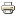# Anti-Conformism in the threshold Model of Collective BehaviorWorking paper
Author/s:
Michel Grabisch, Fen Li
Issue number:
No. 10-2019, January 2019
Series:
ExSIDE Working Paper Series
Year:
2019
We provide a first study of the threshold model, where both conformist and anti-conformist agents coexist. The paper is in the line of a previous work by the first author (Grabisch et al., 2018), whose results will be used at some point in the present paper. Our study bears essentially in answering the following question: Given a society of agents with a certain topology of the network linking these agents, given a mechanism of influence for each agent, how the behavior/opinion of the agents will evolve with time, and in particular can it be expected that it converges to some stable situation, and in this case, which one? Also, we are interested by the existence of cascade effects, as this may constitute a undesirable phenomenon in collective behavior. We divide our study into two parts. In the first one, we basically study the threshold model supposing a fixed complete network, where every one is connected to every one, like in the work of Granovetter (1978). We study the case of a uniform distribution of the threshold, of a Gaussian distribution, and finally give a result for arbitrary distributions, supposing there is one type of anti-conformist. In a second part, the graph is no more complete and we suppose that the neighborhood of an agent is random, drawn at each time step from a distribution. We distinguish the case where the degree (number of links) of an agent is fixed, and where there is an arbitrary degree distribution.
Developed by Paolo Gittoi